Search

About 877 Search Results Matching Types of Worksheet, Worksheet Section, Generator, Generator Section, Grades matching 2nd Grade, Similar to Jonah Crafts for Kids, Page 4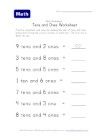Counting Tens and Ones Worksheet

Each question tells kids the number of groups of t...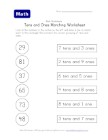Tens and Ones Matching Worksheet - Two of Two

This place value worksheet asks kids to match the ...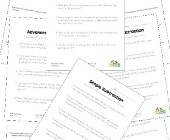Subtraction Word Problems

We have several subtraction word problem worksheet...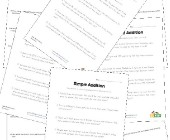We have several addition word problem worksheets t...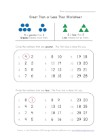Less and Greater Worksheet Two

One section asks kids to find the numbers that are...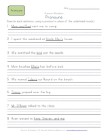Use Pronouns in Sentences - 2nd Grade Pronoun Worksheet 3

Practice using pronouns in sentences in this works...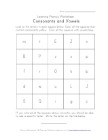Consonant and Vowels Hidden Letter Worksheet

Kids are asked to color the consonant and vowels w...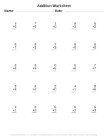This section of addition math worksheets focuses o...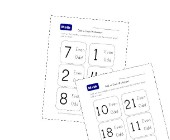Odd and Even Number Recognition

These simple odd and even number recognition works...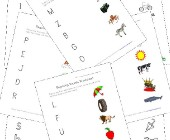Matching Beginning Sounds Worksheets

This group of beginning sounds phonics pages asks ...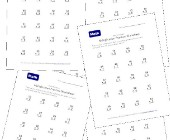Multiplication Practice Worksheets

This section of multiplication math worksheets foc...